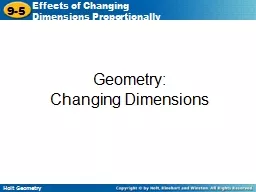# Geometry:2016-06-23 50K 50 0 0

Embed code:

## Geometry:

Download Presentation - The PPT/PDF document "Geometry:" is the property of its rightful owner. Permission is granted to download and print the materials on this web site for personal, non-commercial use only, and to display it on your personal computer provided you do not modify the materials and that you retain all copyright notices contained in the materials. By downloading content from our website, you accept the terms of this agreement.

### Presentations text content in Geometry:

Slide1

Geometry:

Changing Dimensions

Slide2

8th Grade:8.4.10A Describe the resulting effects on perimeter and area when dimensions of a shape are changed proportionally. 8.4.10B Describe the resulting effects on volume when dimensions of a solid figure are changed proportionally.

Student Expectations

Slide3

Describe the effect of each change on the area of the given figure.

Example 1: Effects of Changing One Dimension

The height of the triangle is multiplied by 6.

original dimensions: multiply the height by 6:

Notice that 180 = 6(30). If the height is multiplied by 6, the area is also multiplied by 6.

= 30 in

2

= 180 in2

Slide4

Example 1B: Effects of Changing One Dimension

original dimensions:

The diagonal

SU

of the kite with vertices

R

(2, 2), S(4, 0), T(2, –2), and U(–5,0) is multiplied by .

Slide5

Check It Out! Example 1

The height of the rectangle is tripled. Describe the effect on the area.

A

= bh = (7)(4)

A = bh = (7)(12)

= 28 ft2

= 84 ft2

Notice that 84 = 3(28). If the height is multiplied by 3, the area is tripled.

original dimensions:

triple the height:

Slide6

If the radius of a circle or the side length of a square is changed, the size of the entire figure changes proportionally.

Slide7

Describe the effect of each change on the perimeter or circumference and the area of the given figures.

Example 2A: Effects of Changing Dimensions Proportionally

The base and height of a rectangle with base 4 ft and height 5 ft are both doubled.

Slide8

Example 2B: Effects of Changing Dimensions Proportionally

C = 2(10) = 20 cm

C = 2r

A = (10)2 = 100 cm2

original dimensions:

C = 2(2) = 4 cm

A = (2)2 = 4 cm2

A = r2

J is multiplied by .

dimensions multiplied by .

Slide9

Example 2B Continued

The area is multiplied by

The circumference is multiplied by .

Slide10

Check It Out! Example 2

The base and height of the triangle with vertices P(2, 5), Q(2, 1), and R(7, 1) are tripled. Describe the effect on its area and perimeter.

The perimeter is tripled, and the area is multiplied by 9.

original dimensions:

dimensions tripled:

Slide11

When the dimensions of a figure are changed proportionally, the figure will be similar to the original figure.

Slide12

Example 3A: Effects of Changing Area

A circle has a circumference of 32 in. If the area is multiplied by 4, what happens to the radius?

r2 = 1024

r2 = 1024

r

= √1024 = 32

Set the new area equal to

r2.

Divide both sides by .

Take the square root of both sides and simplify.

and the area is

A = r2 = 256 in2. If the area is multiplied by 4, the new area is 1024 in2.

Notice that 32

= 2(16

). The radius is multiplied by 2.

Slide13

Example 3B: Effects of Changing Area

Let s be a side length of an equilateral triangle. Draw a segment that bisects the top angle and the base to form a 30-60-90 triangle.

An equilateral triangle has a perimeter of 21m. If the area is multiplied by , what happens to the side length?

.

Slide14

Example 3B Continued

The length of each side is , and the area

of the equilateral triangle is

If the area is multiplied by , the new area is

Slide15

Example 3B Continued

Slide16

Check It Out! Example 3

A square has a perimeter of 36 mm. If the area is multiplied by , what happens to the side length?

Slide17

Explain why the graph is misleading.

Example 4: Entertainment Application

The height of the bar representing sales in 2000 is about 2.5 times the height of the bar representing sales in 2003.

Slide18

Example 4 Continued

This means that the area of the bar multiplied by about 2.5

2, or 6.25, so the area of the larger bar is about 6.25 times the area of the smaller bar.

The graph gives the misleading impression that the number of sales in 2003 decreased by 6 times the sales in 2000, but the decrease was actually closer to 2.5 times.

Slide19

Check It Out! Example 4

Use the information in example 4 to create a version of the graph that is not misleading.

Slide20

Lesson Quiz: Part I

Describe the effect of each change on the area of the given figure.

The area is multiplied by 8.

1. The base length of the rectangle is multiplied by 8.

The area is multiplied by 9.

2.

The radius of the circle is tripled.

Slide21

Lesson Quiz: Part II

The side length is doubled.

3. A square has an area of 49 cm2. If the area is quadrupled, what happens to the side length?

4. Rob had a 10 ft by 12 ft wall painted. For a wall twice as wide, the painter charged him twice as much. Is this reasonable? Explain.

Yes; the second wall has twice the area of the first wall.

Slide22

Slide23

Slide24

Slide25

Slide26